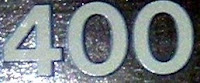## Monday, August 23, 2010

### 400

400 = 24 x 52.

400 = 202, a perfect square.

400 = 70 + 71 + 72 + 73. Hence, 400 is 1111 in base 7.

400 has a representation as a sum of two squares: 400 = 122 + 162.

A full circle is 360 degrees or 400 grads.Years divisible by 400 are leap years, following adoption of the current Gregorian calendar.

Source: Numeropedia